# The Right Distance Approximation in High Dimensions — Fractional distances

The curse of dimensionality is something we all have heard of w.r.t to machine learning.The Sparseness in the vector space being one of the biggest pithole.In this article we will see how the distance metrics behave absurdly in high dimensional space and why clustering these high dimensional vectors can lead to misleading results.Then we will look at what should be the right metric to be used.

Proximity : The concept of proximity or neares neighbour is not quantitatively meaningful when we talk about high dimensions.Generally L2 norm the euclidean is preferred over L1 norm for high dimensional data mining applications.

The Problem : the ratio of the distances of the nearest and farthest neighbors to a given target in high dimensional space is almost 1 for a wide variety of data distributions and distance functions. In such a case, the nearest neighbor problem becomes ill defined, since the contrast between the distances to different data points does not exist. In such cases, even the concept of proximity may not be meaningful from a qualitative perspective: a problem which is even more fundamental than the performance degradation of high dimensional algorithms

where x,y belong to d dimensional space and k is the value of the norm belonging to a set Z. The value of k = 2 makes it euclidean and k = 1 makes it manhattan distance.

From recent research it has been shown that , the fractional value of k works pretty better to define proximity measures in higher dimensions.

Let’s look at the behaviour of the distance metrics :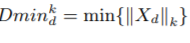Distance of the nearest point from the origin out of all N points.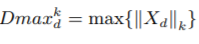Distance of the farthest point from the origin out of all N points.

Don’t be scared looking at the above expression.The above statement is just trying to imply that the difference between the maximum and minimum distances to a given query point does not increase as fast as the nearest distance to any point in high dimensional space. This makes a proximity query meaningless and unstable because there is poor discrimination between the nearest and furthest neighbor.

It is called the relative contrast. It has been used to define meaningfulness of distance metric.

Result from the above theorem :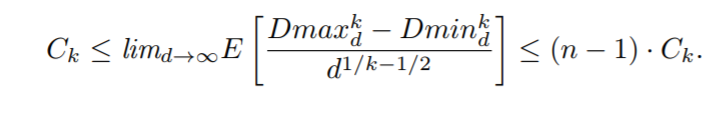here Ck is some arbitrary constant

The result shows that in high dimensional space Dmaxk d − Dmink d increases at the rate of d1/k−1/2, independent of the data distribution. This means that for the manhattan distance metric, the value of this expression diverges to ∞; for the Euclidean distance metric, the expression is bounded by constants whereas for all other distance metrics. Furthermore, the convergence is faster when the value of k of the Lk metric increases. This provides the insight that higher norm parameters provide poorer contrast between the furthest and nearest neighbor. Even more insight may be obtained by examining the exact behavior of the relative contrast as opposed to the absolute distance between the furthest and nearest point.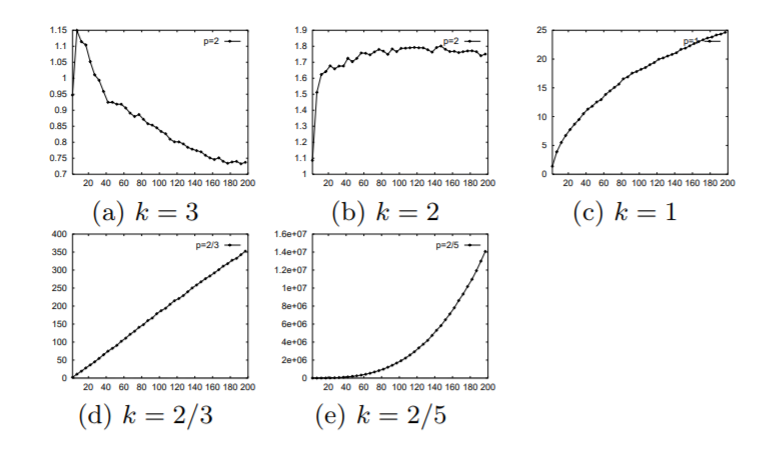plot with x axis dimensionality of data and y axis as the difference between dmax and dmin

We see in the above plots for norms between k = 2/3 to k = 3 that the difference decreases for k =3 and it is significant in the case of k =2/3.

Another interesting aspect which can be explored to improve nearest neighbor and clustering algorithms in high-dimensional space is the effect of k on the relative contrast. Even though the expected relative contrast always decreases with increasing dimensionality, this may not necessarily be true for a given data set and different k.

The experiment observation showed that value of contrast decreases for Euclidean compared to Manhattan when we increase the dimensions.

Thus,for higher dimensionality, the relative contrast provided by a norm with smaller parameter k is more likely to dominate another with a larger parameter

FRACTIONAL DISTANCE METRICS:

After observing that fractional distances work better with proximity measurement , Let’s see, how exactly and why these fractional distances better.

A fractional distance is defined by Lf norm where f belongs to (0,1)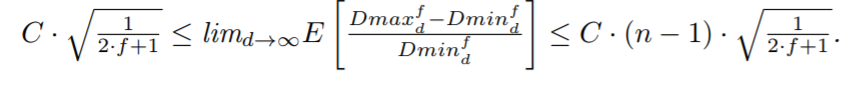Contrast Estimation range for fractional distances

where n is the number of data points from normal distribution, f is the norm value between 0 and 1.

fractional distance metrics provide better contrast than integral distance metrics both in terms of the absolute distributions of points to a given query point and relative distances. This is a surprising result in light of the fact that the Euclidean distance metric is traditionally used in a large variety of indexing structures and data mining applications. The widespread use of the Euclidean distance metric stems from the natural extension of applicability to spatial database systems (many multidimensional indexing structures were initially proposed in the context of spatial systems). However, from the perspective of high dimensional data mining applications, this natural interpretability in 2 or 3-dimensional spatial systems is completely irrelevant.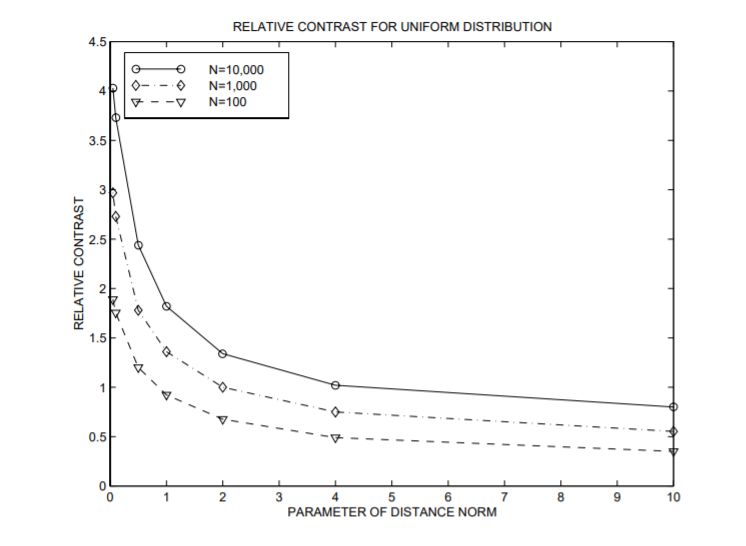Relative contrast variation with norm parameter for the uniform distribution

The curve for each value of N(number of data points drawn from a distribution) is different but all curves fit the general trend of reduced contrast with increased value of f. Note that the value of the relative contrast for both, the case of integral distance metric Lk and fractional distance metric Lf is the same in the boundary case when f = k = 1.

Conclusion:

Maybe instead of using L2 norm to define distance for our high dimensional data, we should be opting for fractional norm to have better relative contrast between the data points which makes proximity more meaningful in higher dimensions.

References for graphs and plots :